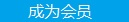您可以捐助，支持我们的公益事业。 1元 10元 50元 认证码：必填求知 文章 文库 Lib 视频 Code iProcess 课程 角色 咨询 工具 火云堂 讲座吧 建模者要资料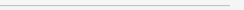订阅捐助
python机器学习之神经网络实现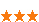433 次浏览     评价： 好 中 差
2018-10-25

 编辑推荐: 本文来自于个人博客，这篇文章主要为大家详细介绍了python机器学习之神经网络的实现方法。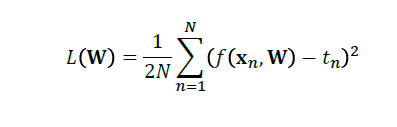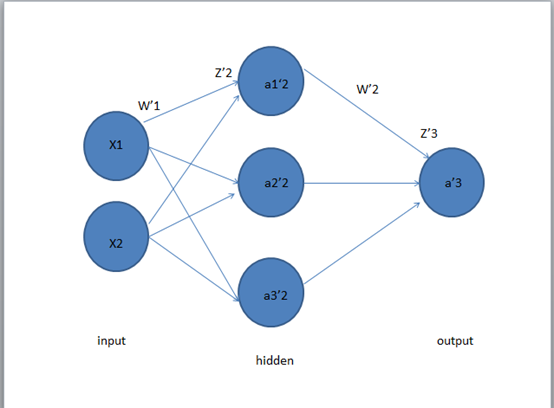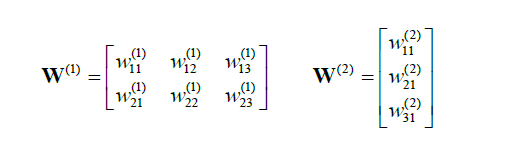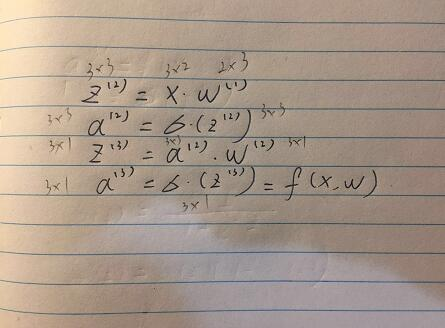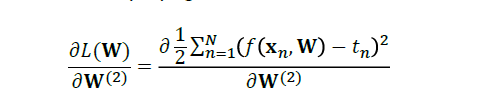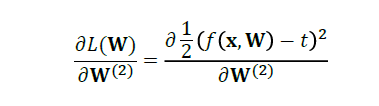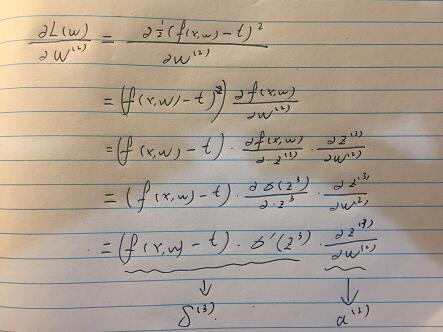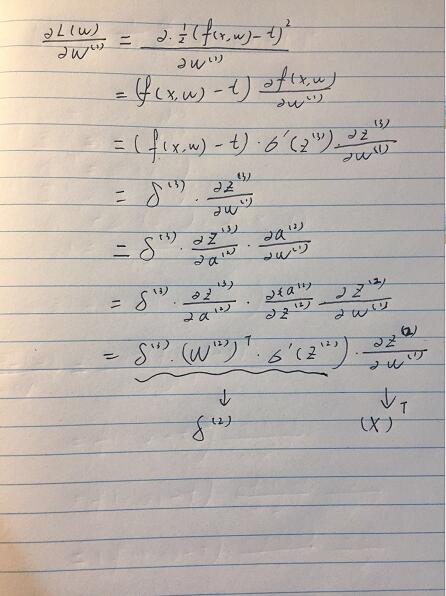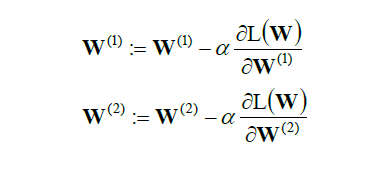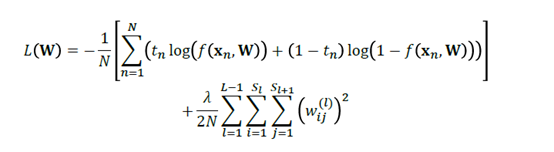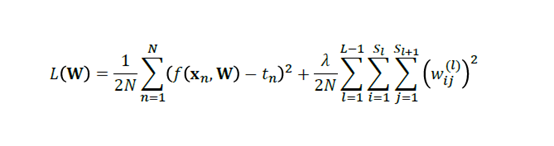Python 的实现：

 model.add(Dense(1, input_dim=n_features, activation='linear', use_bias=True)) # Use mean squared error for the loss metric and use the ADAM backprop algorithm model.compile(loss='mean_squared_error', optimizer='adam') # Train the network (learn the weights) # We need to convert from DataFrame to NumpyArray history = model.fit(X_train.values, y_train.values, epochs=100, batch_size=1, verbose=2, validation_split=0)

 # create model model = Sequential() model.add(Dense(64, activation='relu', input_dim=n_features)) model.add(Dropout(0.5)) model.add(Dense(64, activation='relu')) model.add(Dropout(0.5)) # Softmax output layer model.add(Dense(7, activation='softmax')) model.compile(loss='categorical_crossentropy', optimizer='adam', metrics=['accuracy']) model.fit(X_train.values, y_train.values, epochs=20, batch_size=16) y_pred = model.predict(X_test.values) y_te = np.argmax(y_test.values, axis = 1) y_pr = np.argmax(y_pred, axis = 1) print(np.unique(y_pr)) print(classification_report(y_te, y_pr)) print(confusion_matrix(y_te, y_pr))

 from sklearn.model_selection import GridSearchCV def create_model(optimizer='rmsprop'): model = Sequential() model.add(Dense(64, activation='relu', input_dim=n_features)) model.add(Dropout(0.5)) model.add(Dense(64, activation='relu')) model.add(Dropout(0.5)) model.add(Dense(7, activation='softmax')) model.compile(loss='categorical_crossentropy', optimizer=optimizer, metrics=['accuracy']) return model model = KerasClassifier(build_fn=create_model, verbose=0) optimizers = ['rmsprop'] epochs = [5, 10, 15] batches =  param_grid = dict(optimizer=optimizers, epochs=epochs, batch_size=batches, verbose=['2']) grid = GridSearchCV(estimator=model, param_grid=param_grid) grid.fit(X_train.values, y_train.values)433 次浏览  评价: 好 中 差订阅捐助
 相关文章 我们该如何设计数据库 数据库设计经验谈 数据库设计过程 数据库编程总结
 相关文档 数据库性能调优技巧 数据库性能调整 数据库性能优化讲座 数据库系统性能调优系列每天2个文档/视频 扫描微信二维码订阅
 订阅技术月刊 获得每月300个技术资源